International
Tables for
Crystallography
Volume D
Physical properties of crystals
Edited by A. Authier

International Tables for Crystallography (2013). Vol. D, ch. 1.10, p. 259

## Section 1.10.4.6.5. Elasticity tensor for an icosahedral quasicrystal

T. Janssena*

aInstitute for Theoretical Physics, University of Nijmegen, 6524 ED Nijmegen, The Netherlands
Correspondence e-mail: ted@sci.kun.nl

#### 1.10.4.6.5. Elasticity tensor for an icosahedral quasicrystal

| top | pdf |

The point group of an icosahedral quasicrystal is 532(5232) with generators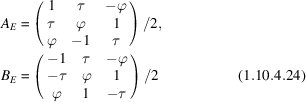in physical space, with,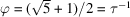, and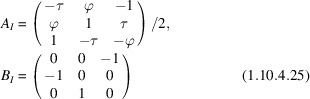in internal space (c.f. Table 1.10.5.2). The phonon and phason strain tensors form a 6D, respectively 9D, vector space. The generators of the superspace point group act in these spaces as follows.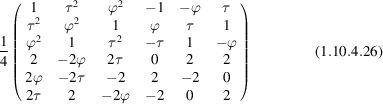for the first generator in the 6D space,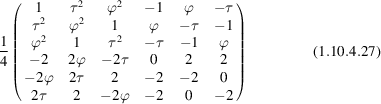for the second generator in the 6D space,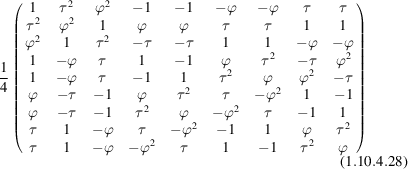for the first generator in the 9D space and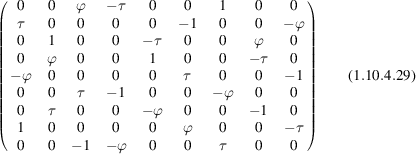for the second generator in the 9D space.

This implies that the phonon elasticity tensors form a 21D space, the phason elasticity tensors a 45D space and the phonon–phason coupling a 54D space. The invariant vectors under these orthogonal transformations correspond to invariant elastic tensors. Their coordinates are the elastic constants. For the given presentation of the point group, these are given in Table 1.10.4.3. The tensor elements are expressed in parameters x and y where there are two independent tensor elements. The tensor elements that are not given are zero or equal to that given by the permutation symmetry. If bases for the phonon and phason strain are introduced byfor the phonon part andfor the phason part, the elastic tensors may be given in matrix form asTable 1.10.4.3| top | pdf | Elastic constants for icosahedral quasicrystals
TypeFree parametersRelations
Phonon–phonon 2Phason–phason 2Phonon–phason 1The parameters x, y, z, u, v are the five independent elastic constants.